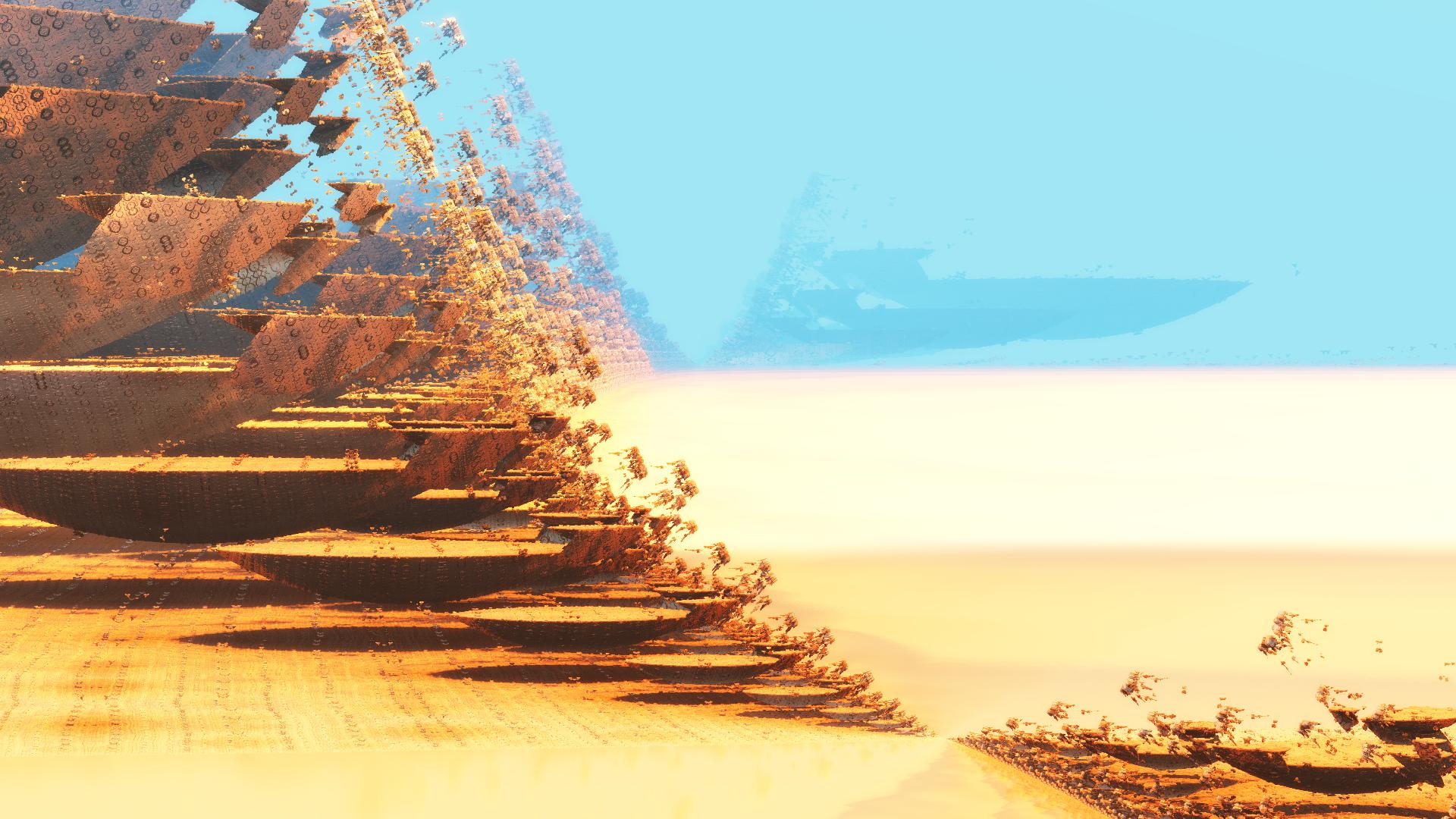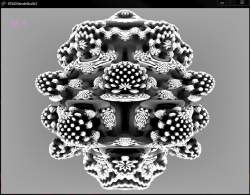News: Follow us on Twitter## The All New FractalForums is now in Public Beta Testing! Visit FractalForums.org and check it out!

Solar sail test failPrevious Image | Next Image
Description: Fragementarium-v1.0.27 , Kali's Asurf, DE-Kn8 ..

Made me think of a failed testing of the microsats planned to be boosted on intergalactic travel by high intensity laser pulses.

#info Amazing Surface by Kali - Based on Tglad's Amazing Box
#define providesInit
#include "DE-Kn8.frag"

#define KN_VOLUMETRIC
#define USE_IQ_CLOUDS

#include "MathUtils.frag"

#define USE_INF_NORM
#group AmazingSurface

uniform int Iterations;  slider[0,17,300]
uniform int ColorIterations;  slider[0,3,300]
uniform float Scale;  slider[-3,1.5,3.0]
uniform int FoldType; slider[1,1,3]
uniform vec3 PreTranslation; slider[(-5,-5,-5),(0,0,0),(5,5,5)]
uniform vec2 FoldValues; slider[(0,0),(1,1),(5,5)]
uniform bool Julia; checkbox[false]
uniform vec3 JuliaValues; slider[(-5,-5,-5),(-1,-1,-1),(5,5,5)]
uniform vec3 RotVector; slider[(-1,-1,-1),(1,1,1),(1,1,1)]
uniform float RotAngle; slider[-180,0,180]

mat3 rot;

void init() {
rot = rotationMatrix3(normalize(RotVector), RotAngle);
}

vec4 scale = vec4(Scale, Scale, Scale, abs(Scale))/MinRad2;
float absScalem1 = abs(1-Scale);
float AbsScaleRaisedTo1mIters = pow(abs(Scale), float(1-Iterations));

float DE(vec3 pos) {
vec4 p = vec4(pos,1);
vec3 c = Julia?JuliaValues:p.xyz;
for (int i=0; i<Iterations; i++) {
if (FoldType==1) p.xy=abs(p.xy+FoldValues)-abs(p.xy-FoldValues)-p.xy;
if (FoldType==2) p.xy=FoldValues-abs(abs(p.xy)-FoldValues);
if (FoldType==3) p.xy=abs(p.xy+FoldValues);
p.xyz+=PreTranslation;
float r2 = dot(p.xyz, p.xyz);
if (i<ColorIterations) orbitTrap = min(orbitTrap, abs(vec4(p.xyz,r2)));
p = p*scale;
p.xyz+=c;
p.xyz*=rot;
}
return ((length(p.xyz) - absScalem1) / p.w - AbsScaleRaisedTo1mIters);
}

#preset timemit
FOV = 0.1277174
Eye = 7.947994,-1.131831,0.7783462
Target = 7.907182,41.28708,2.020285
Up = -0.0155932,-1,-1
Gamma = 0.8999
ToneMapping = 5
Exposure = 0.45597
Brightness = 1.281513
Contrast = 2.22735
Saturation = 3.7337
GaussianWeight = 1
AntiAliasScale = 0.7129
Detail = -3.62453
FudgeFactor = 1
Dither = 0.43043
NormalBackStep = 5.957
AO = 0,0,0,1
CamLight = 0.811765,0.909804,0.937255,1.538136
CamLightMin = 0.424581
Glow = 1,0.721569,0.490196,0.31962
GlowMax = 479
BaseColor = 1,1,1
OrbitStrength = 0.60397
X = 0.105882,0.129412,0.129412,-0.01108
Y = 0.45098,0.364706,0.219608,1
Z = 0.827451,0.764706,0.772549,-0.04244
R = 0.87451,0.858824,0.67451,0.36842
BackgroundColor = 0.921569,0.811765,0.168627
CycleColors = true
Cycles = 9.60898
EnableFloor = true NotLocked
FloorNormal = 0.0174703,-0.0356394,-1
FloorHeight = 0.2896022
FloorColor = 0.878431,0.968627,1
FocalPlane = 2.54299
Aperture = 0.07814
InFocusAWidth = 1
ApertureNbrSides = 5 NotLocked
ApertureRot = 136.325
ApStarShaped = false NotLocked
ReflectionsNumber = 3
Bloom = true
BloomIntensity = 0.2117982
BloomPow = 1.832865
BloomTaps = 17
DetailAO = -1.75329
MaxRaySteps = 921
MaxDistance = 567.12
AoCorrect = 0.4650035
Specular = 0.0317023
SpecularExp = 16
Reflection = 0.2313725,0.172549,0.145098
SpotGlow = true
SpotLight = 1,0.631373,0.109804,3.704755
LightPos = 7.628294,-0.8044383,-0.0970874
LightSize = 0.1862069
LightFallOff = 0.4704245
LightGlowExp = 0.4166667
HF_Fallof = 2.021722
HF_Const = 0.0512111
HF_Intensity = 0.2363636
HF_Dir = -0.3145604,-0.0824176,0.3928571
HF_Offset = -3.393855
HF_Color = 0.4156863,0.6666667,0.8196078,1.118644
HF_Scatter = 1.6635
HF_Anisotropy = 0,0,0
HF_FogIter = 8
CloudScale = 0.57362
CloudFlatness = 0.12432
CloudTops = -7.8968
CloudBase = -8.9474
CloudDensity = 0.09519
CloudRoughness = 0.5894
CloudContrast = 1.7731
CloudColor = 0.905882,0.886275,0.588235
SunLightColor = 0.698039,0.203922,0.105882
Iterations = 93
ColorIterations = 8
Scale = 1.302277
FoldType = 3
PreTranslation = -1.896797,0.088968,0.1529349
FoldValues = 0.9215137,2.207428
Julia = true
JuliaValues = -3.973457,-5,-0.4716274
RotVector = 0.7798036,0.57223,-0.6970547
RotAngle = -1.015515
#endpreset

Stats:
Total Favorities: 0 View Who Favorited
Filesize: 279.76kB
Height: 1080 Width: 1920
Discussion Topic: View Topic
Keywords: timemit fragmentarium kali amazing surface laser solar sail
Posted by: Tim EmitOctober 13, 2016, 10:30:38 AM

Rating: Has not been rated yet.

 Solar SnowflakeRating:Filesize: 582.44kBDate: August 14, 2009, 07:13:39 PMComments (0)By: Ross Hilbert Mandelbulb ambiant oclusion fail invertedRating:Filesize: 106.65kBDate: August 08, 2010, 12:54:16 AMComments (0)By: knighty Solar Plexus ChakraRating:Filesize: 383.99kBDate: August 13, 2010, 01:57:23 PMComments (0)By: Guest Geodesic omnidirectional solar panels of the GodsRating:Filesize: 431.77kBDate: December 29, 2010, 08:01:08 AMComments (0)By: Guest Solar Nervous SystemRating:Filesize: 360.05kBDate: January 18, 2011, 11:43:35 AMComments (2)By: KRAFTWERK
 Comments (1)Sabine Fractal FertilizerPosts: 373October 14, 2016, 07:36:25 PMYou couldn't have known a sand-storm is coming!Great atmosphere!